# 连续及不连续各向异性热传导问题的数值流形方法求解*

(南昌航空大学 土木建筑学院， 南昌 330063)

## 引 言

20世纪90年代初，由石根华博士创立的数值流形方法诞生于岩石工程领域该方法因数学中“流形”一词而获名，其融合了FEM和非连续变形分析方法(DDA)，可实现对连续和不连续问题的统一求解NMM的主要特点为：1) 数学覆盖(类似于FEM网格)无需适应物理域内外边界形状；2) 在保持原有数学覆盖不变的情况下，采用高阶形式的局部函数便可获得高阶次的整体逼近，以达到提高解精度的目的；3) 通过合理选取局部函数可准确表征待研究问题的局部物理特性；4) 可自然描述某些物理量(如跨越裂纹面的温度场)的不连续性经过多年的发展，NMM已在理论及应用层面取得了较多的研究成果，如文献[8-16]在热分析方面，林绍忠等采用NMM分析了大体积混凝土结构的温度场及温度应力；刘泉声和刘学伟提出了裂隙岩体二维不稳定温度场求解的NMM；谭育新等利用正六边形NMM研究了二维各向同性材料中的稳态热传导问题；Zhang等[20-21]基于NMM对FGM稳态及瞬态热断裂问题进行了研究

## 1 控制方程及边界条件

(kijT, j),i+Q=0，

(1)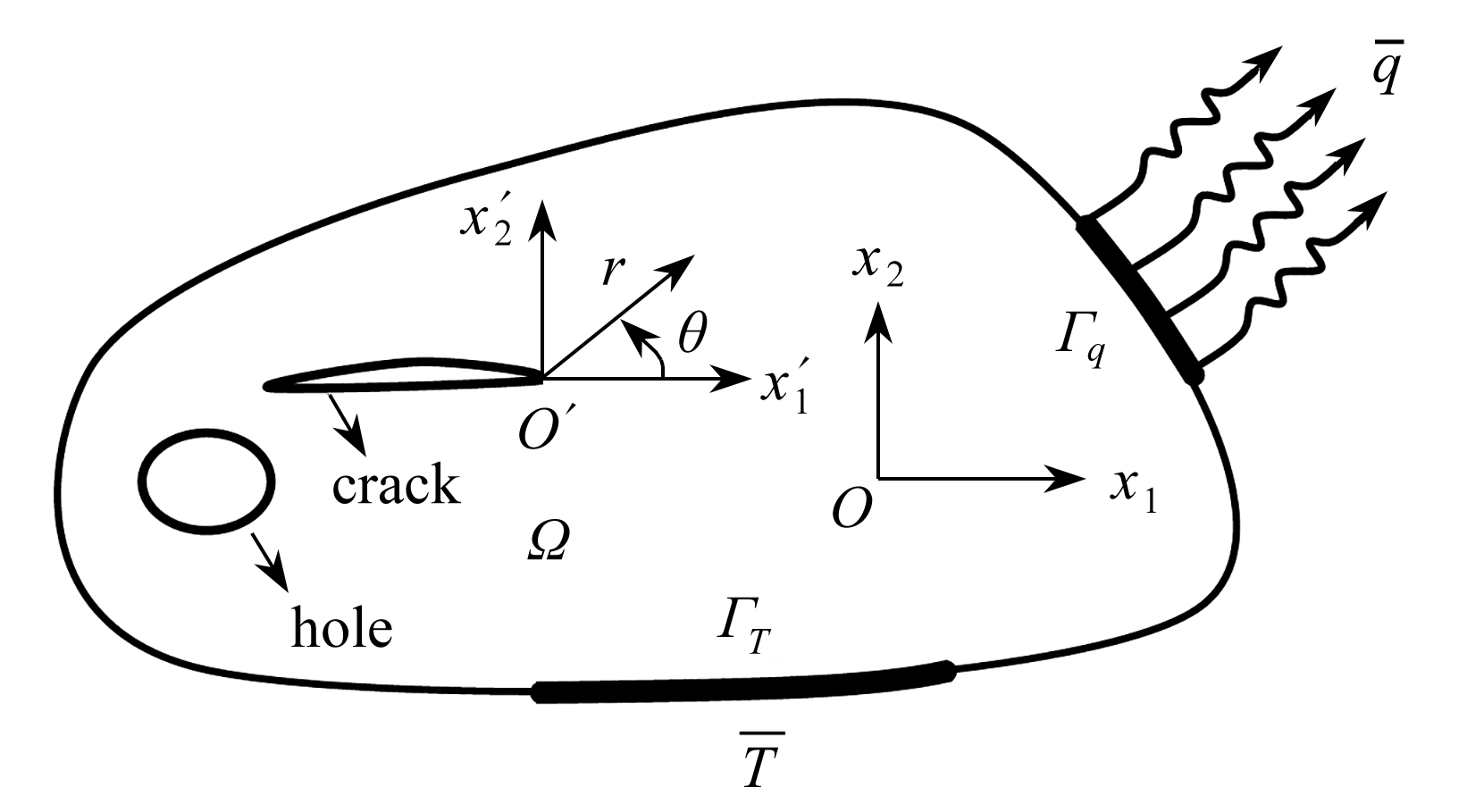Fig. 1 Heat conduction in an anisotropic material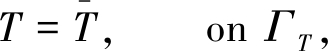(2)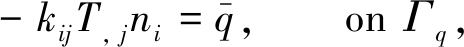(3)

## 2 NMM求解各向异性热传导问题

### 2.1 NMM简介

NMM引入了两套覆盖系统(即数学覆盖和物理覆盖)求解物理问题数学覆盖是由一系列允许相互重叠且形状任意的数学分片组成，无需与物理域的内外边界重合，只需完全覆盖整个物理域物理分片由各个数学分片和物理域求交而成，所有物理分片的并集则组成物理覆盖若干个物理分片的重叠部分便形成NMM的基本计算单元，即流形单元(以下简称单元)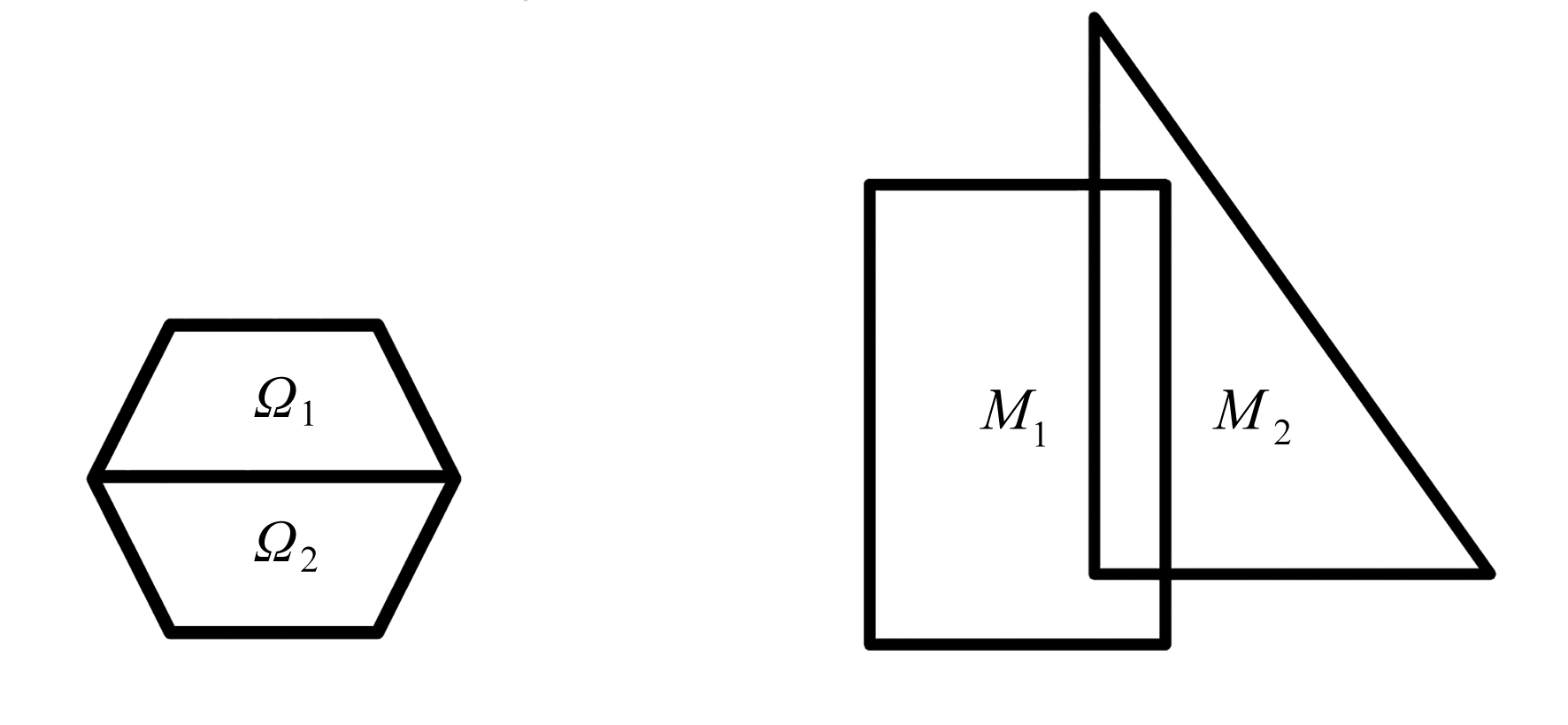(a) 物理域 (b) 数学覆盖
(a) The physical domain(b) The mathematical cover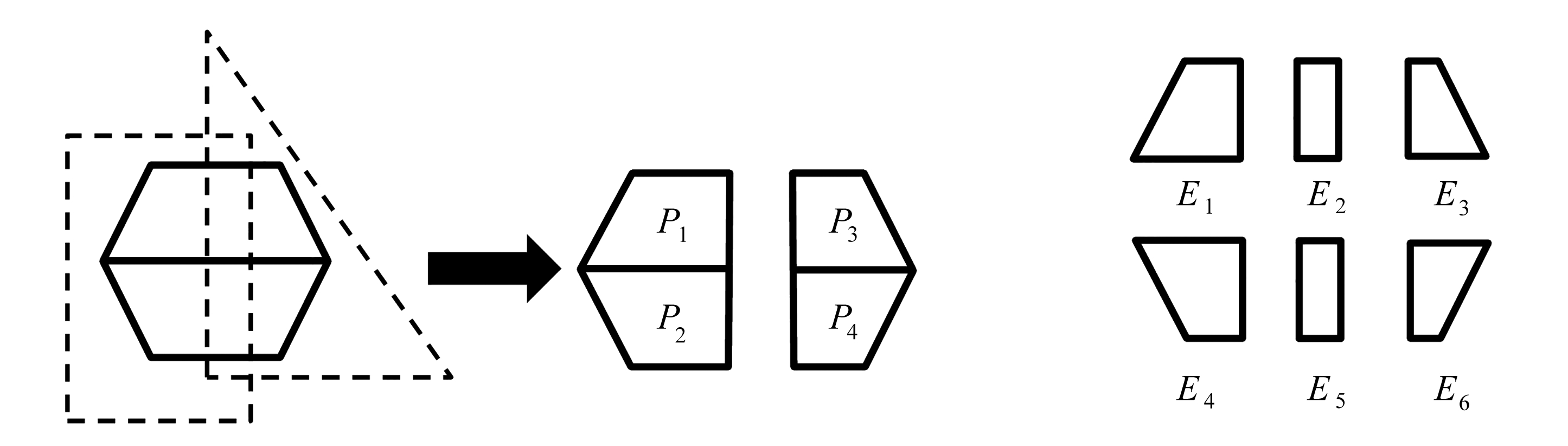(c) 物理分片的形成过程 (d) 单元的生成
(c) Generation of physical patches (d) Generation of manifold elements

Fig. 2 Illustration of the NMM discretization

### 2.2 NMM的温度场近似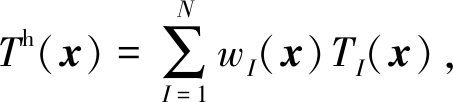(4)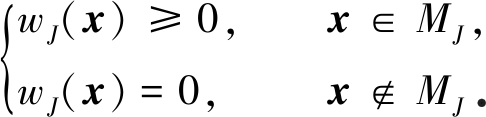(5)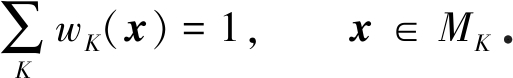(6)

TI(x)为定义在PI上的局部函数，对于连续物理分片(即内部无裂纹等不连续因素的物理分片)，常取为

TI(x)=P(x)aI

(7)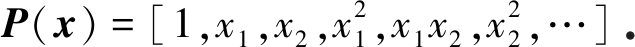(8)

TI(x)=P(x)aI+Φ(x)bI

(9)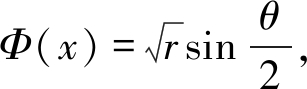(10)

### 2.3 NMM求解各向异性热传导问题的总体方程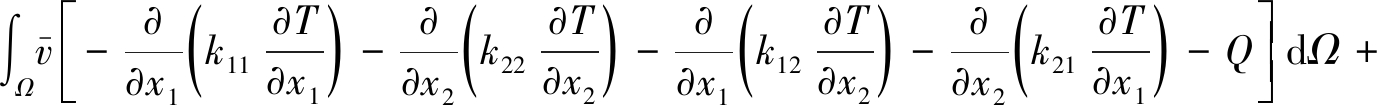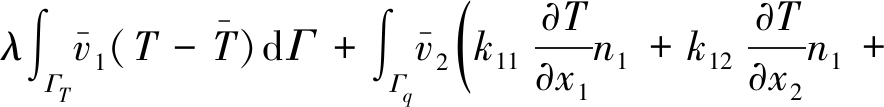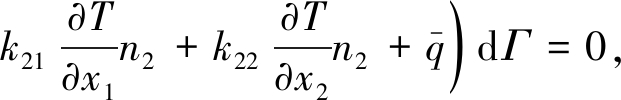(11)(δ为一阶变分)并利用Green公式对式(11)中第一个积分的前四项进行分部积分后，得到其弱形式：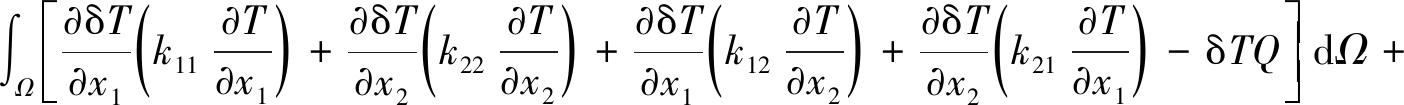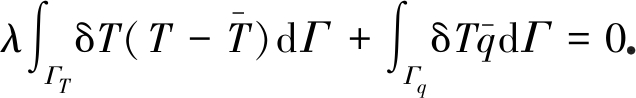(12)

δΠ(T)=0，

(13)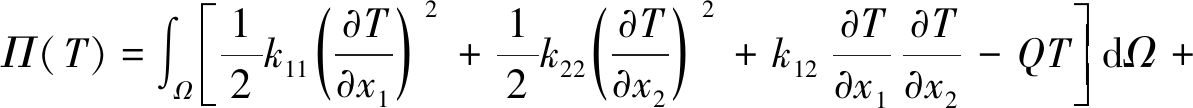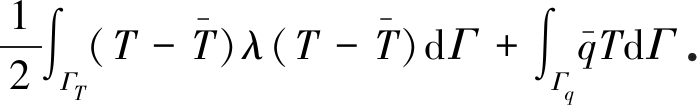(14)

δT≈δTh=wIδTI

(15)

KT=F

(16)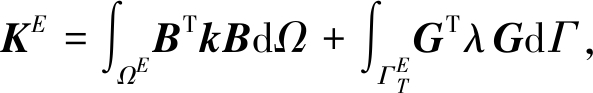(17)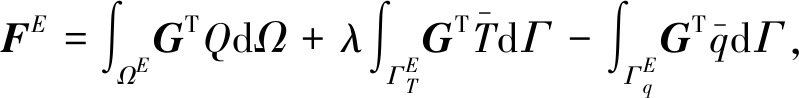(18)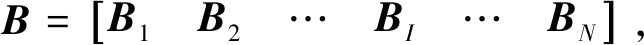(19)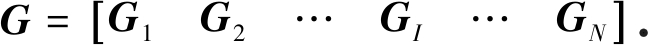(20)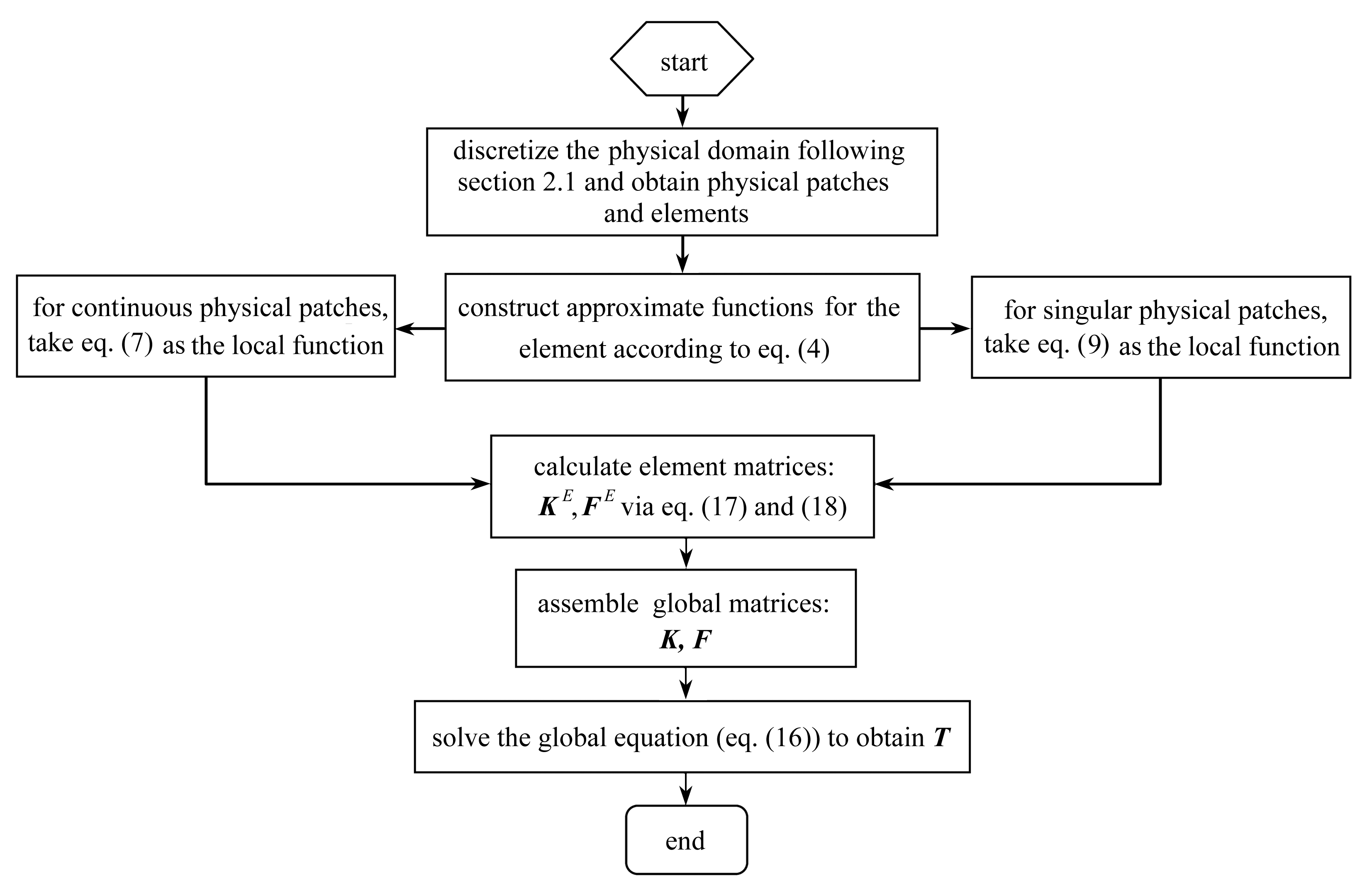Fig. 3 The flowchart of the NMM for heat conduction problems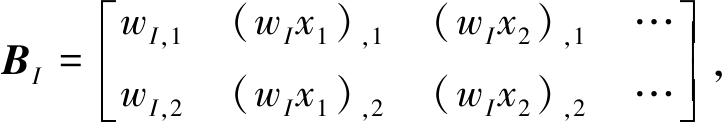(21)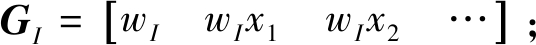(22)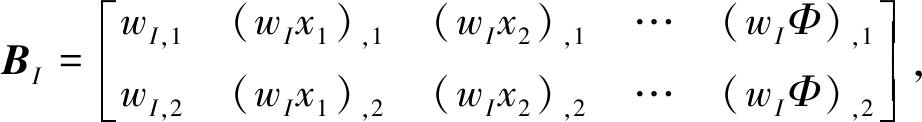(23)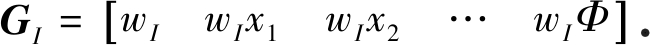(24)

## 3 数 值 算 例

### 3.1 有热源矩形板的热传导问题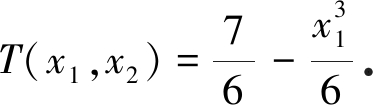(25)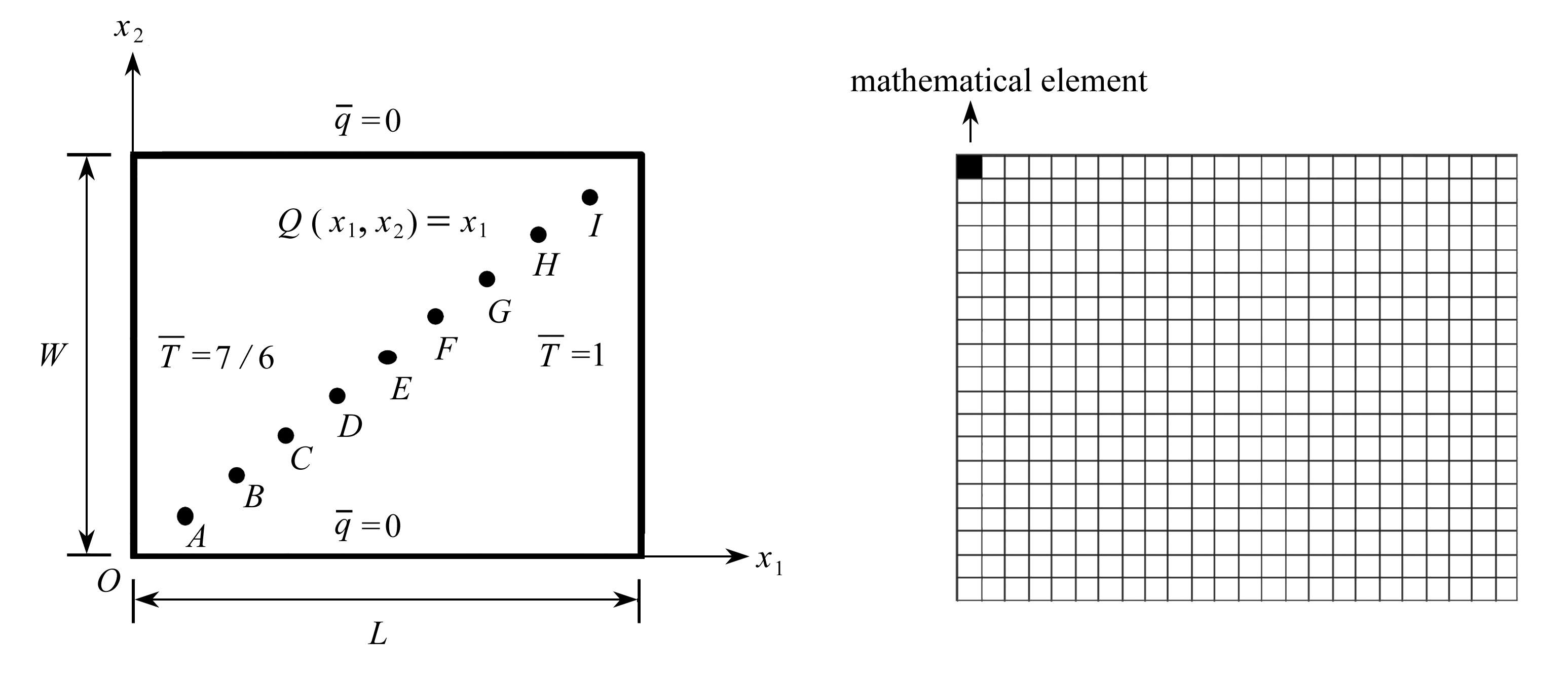Fig. 4 The physical model Fig. 5 The mathematical cover for h=0.05

NMM仿真时，为探讨本文方法的收敛性，选用四种尺寸分别为h=0.4,0.2,0.1,0.05的数学覆盖对平板进行剖分(h为数学单元的边长，以h=0.05的情况为例，其对应的数学覆盖见图5，其中左上角的填充区即为数学单元，四个数学单元则构成一个数学分片)图6给出了h取不同值时的离散域，相应的物理分片和单元数量列于表1图7给出了h=0.05时的温度场分布情况，可以看出，温度场仅沿水平方向变化，此规律与式(21)中解析解的形式能很好地吻合表2列出了h取不同值时九个测点(测点编号及坐标分别为：A(0.1, 0.08)，B(0.2, 0.16)，C(0.3, 0.24)， D(0.4, 0.32)， E(0.5, 0.40)， F(0.6, 0.48)， G(0.7, 0.56)， H(0.8, 0.64)和I(0.9, 0.72))温度的NMM结果及对应的精确解，由表中的数据可知，随着单元尺寸的减小，计算结果逐渐逼近理论解，很好地展示了方法的收敛性及精度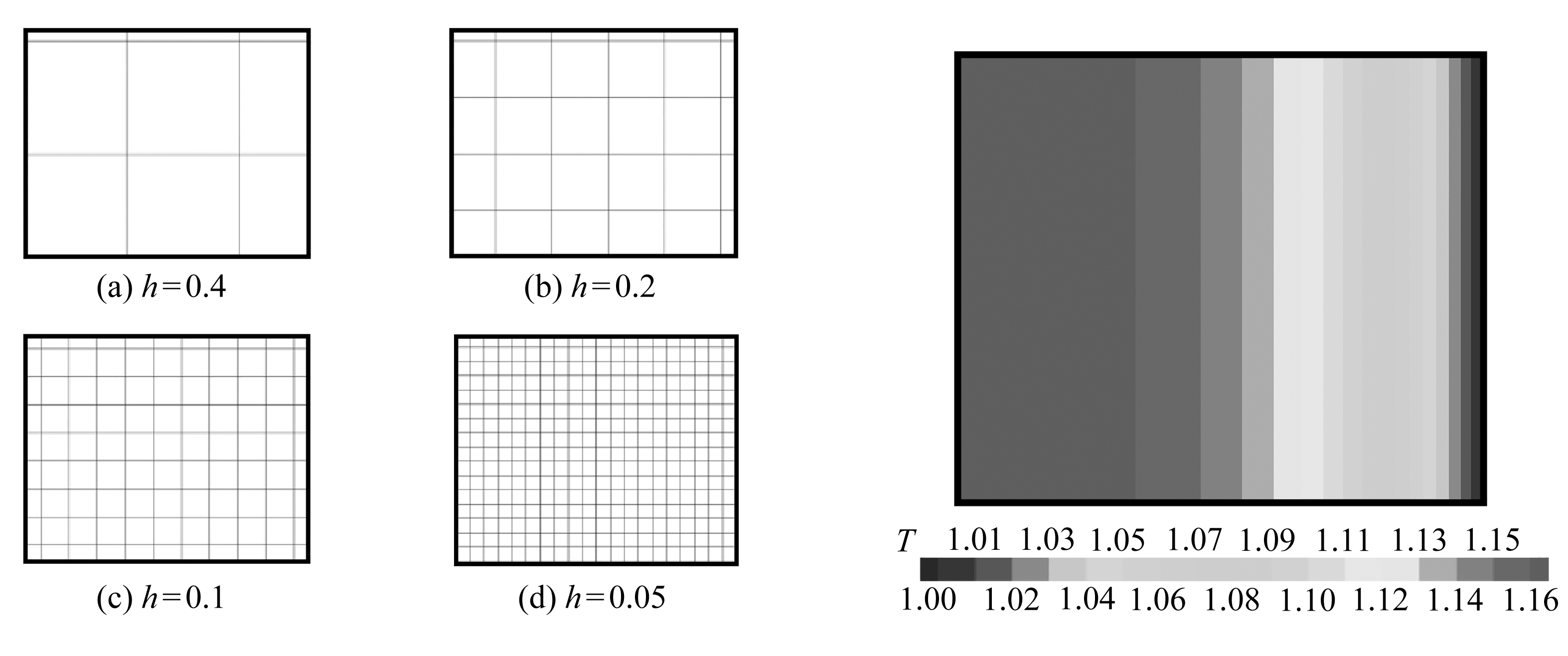Fig. 6 Discretized domains at different Fig. 7 Temperature distribution for h=0.05mathematical element sizes

Table 1 Numbers of physical patches and elements at different mathematical element sizes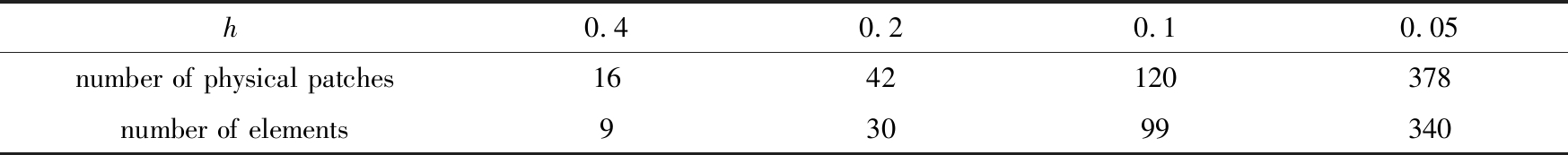h0.40.20.10.05number of physical patches1642120378number of elements93099340

Table 2 Temperatures of measuring points at different mathematical element sizes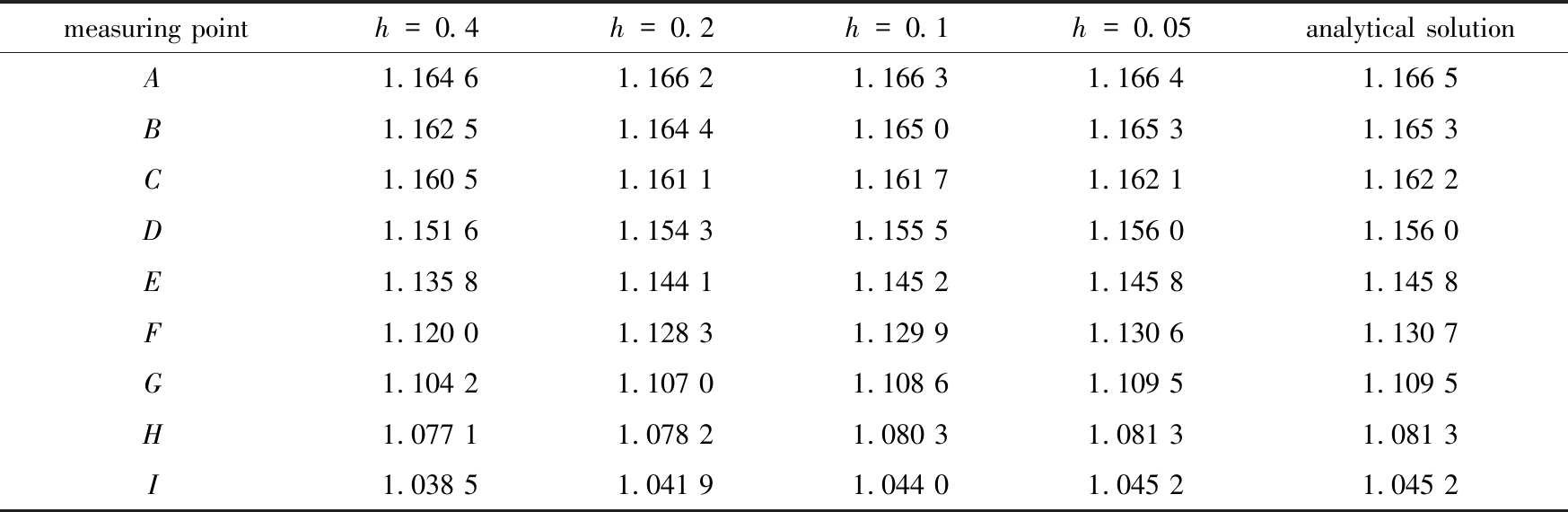measuring pointh=0.4h=0.2h=0.1h=0.05analytical solutionA1.164 61.166 21.166 31.166 41.166 5B1.162 51.164 41.165 01.165 31.165 3C1.160 51.161 11.161 71.162 11.162 2D1.151 61.154 31.155 51.156 01.156 0E1.135 81.144 11.145 21.145 81.145 8F1.120 01.128 31.129 91.130 61.130 7G1.104 21.107 01.108 61.109 51.109 5H1.077 11.078 21.080 31.081 31.081 3I1.038 51.041 91.044 01.045 21.045 2

### 3.2 无热源圆板的热传导问题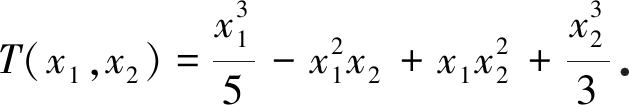(26)

NMM模拟时，采用h=0.04的数学覆盖对板进行剖分，离散后的圆板如图9所示，其中包含2 172个物理分片和2 069个单元图10给出了温度场的整体分布情况表3给出了六个测点(即A(-0.5, 0.75)， B(0.0, 0.75)， C(0.5, 0.75)， D(-0.5, 0.5)， E(0.0, 0.5)和F(0.5, 0.5))温度值的NMM结果及相应的理论解，且两者最大相对误差为1.95%，可见数值解与解析解具有很好的一致性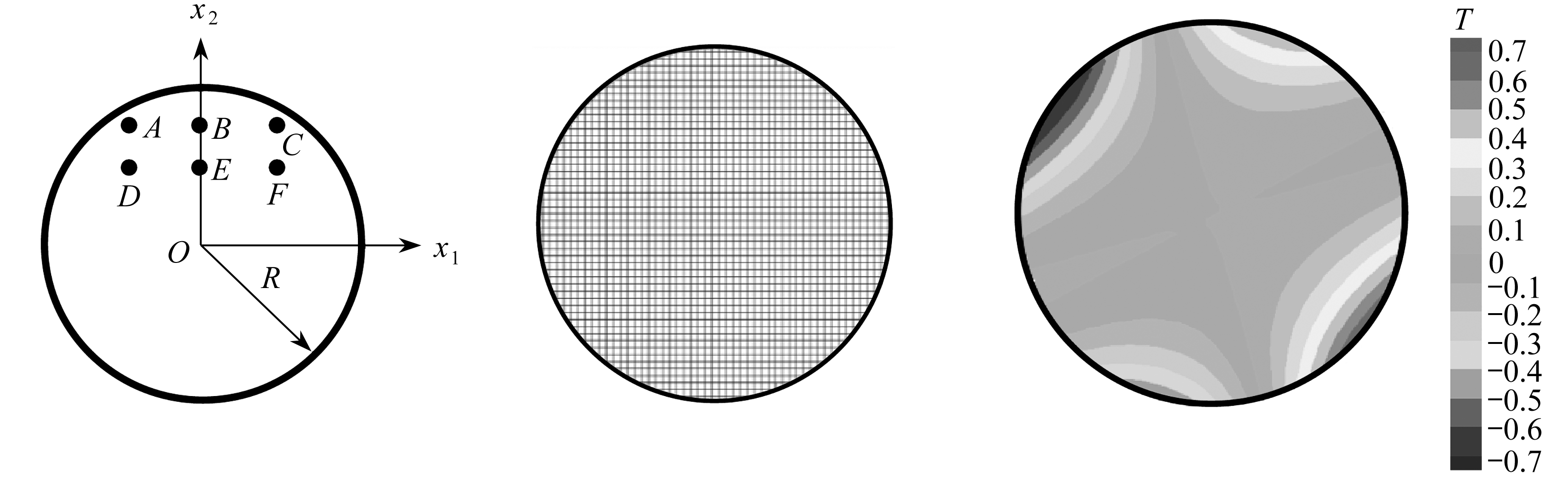Fig. 8 The physical modelFig. 9 The discretized domainFig. 10 The temperature distribution

Table 3 Temperatures at different measuring points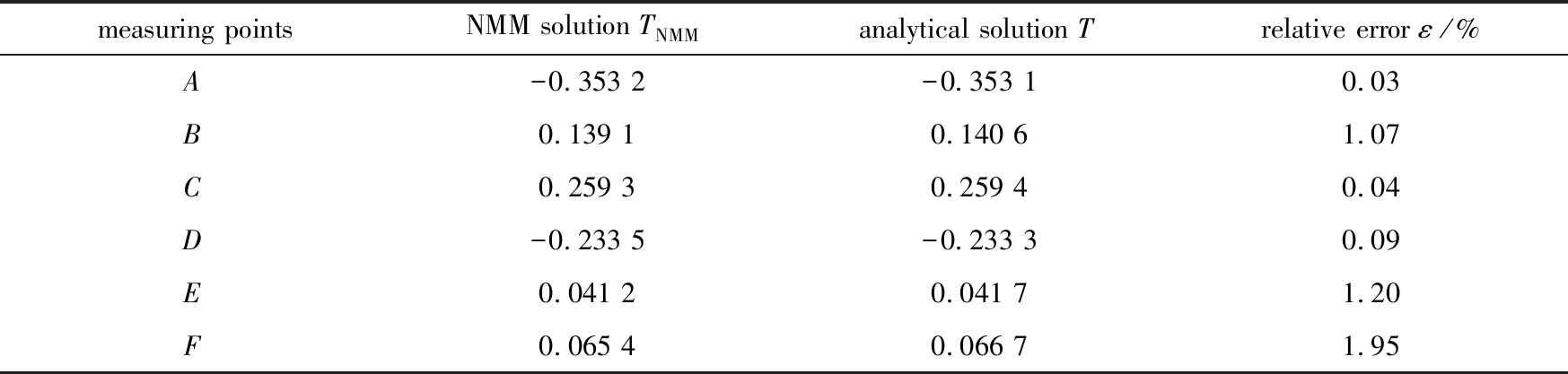measuring pointsNMM solution TNMM analytical solution Trelative error ε/%A-0.353 2-0.353 10.03B0.139 10.140 61.07C0.259 30.259 40.04D-0.233 5-0.233 30.09E0.041 20.041 71.20F0.065 40.066 71.95

### 3.3 含孔洞无热源FGM板的热传导问题

k11=eβx1

(27)

k22=eβx2

(28)

NMM分析时主要考察材料梯度对温度场的影响，为此，分别取β=0.2,0.4,0.6,…,1.2数学单元尺寸h=0.04,对应的离散域见图12，其中包含2 332个物理分片和2 116个单元图13给出了β=1的温度场等值云图，可以发现孔洞的存在对温度场具有明显的扰动作用图14给出了梯度参数取不同值时五个测点：A(-0.5, 0.3)， B(0.3, 0.5)， C(-0.3, -0.5)， D(0.5, -0.3)和E(0.4, 0.0)的温度变化情况，可知各测点的温度均随β的增大而减小

### 3.4 含裂纹无热源FGM板的热传导问题

k11=2x1+8,

(29)

k22=2x2+8

(30)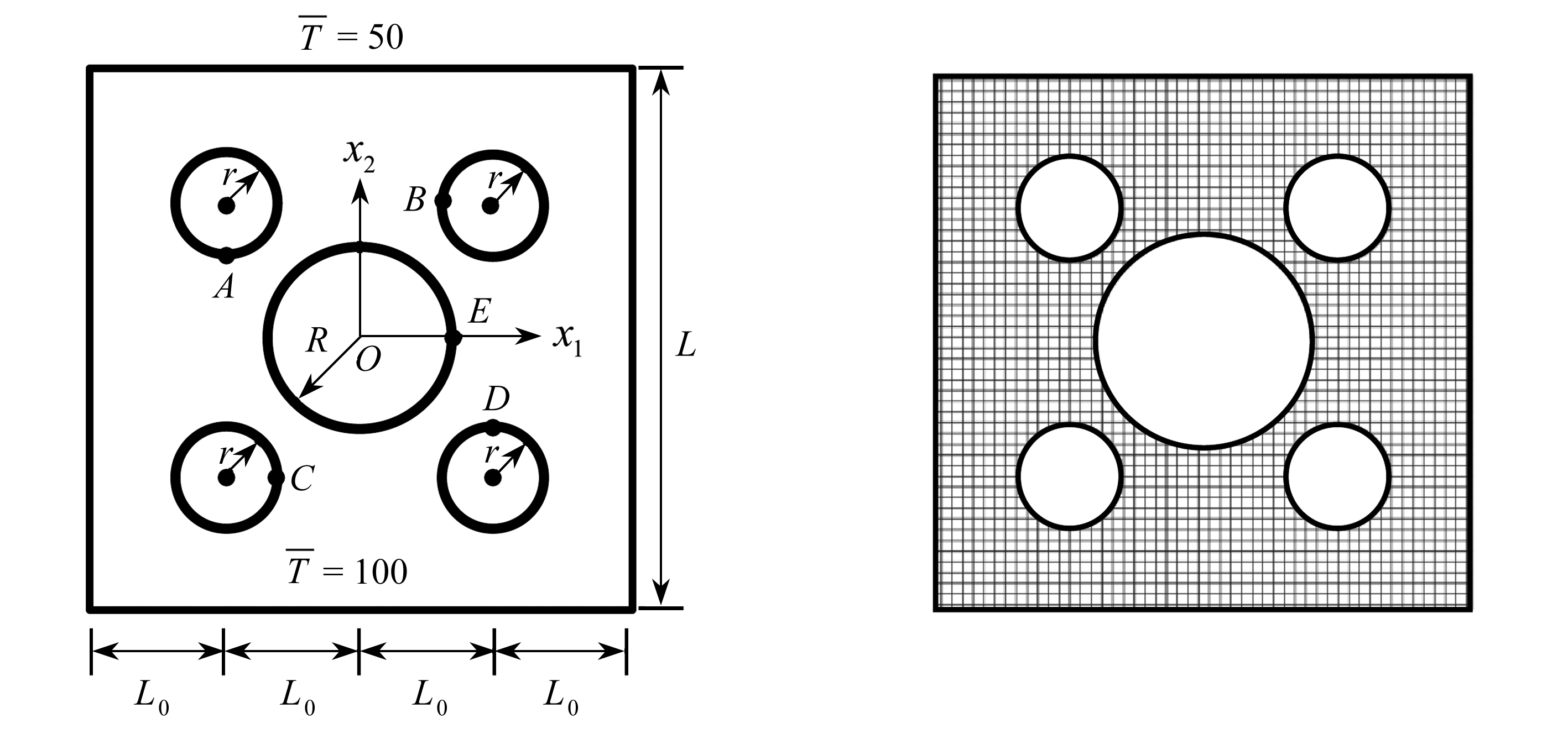Fig. 11 The physical model Fig. 12 The discretized domain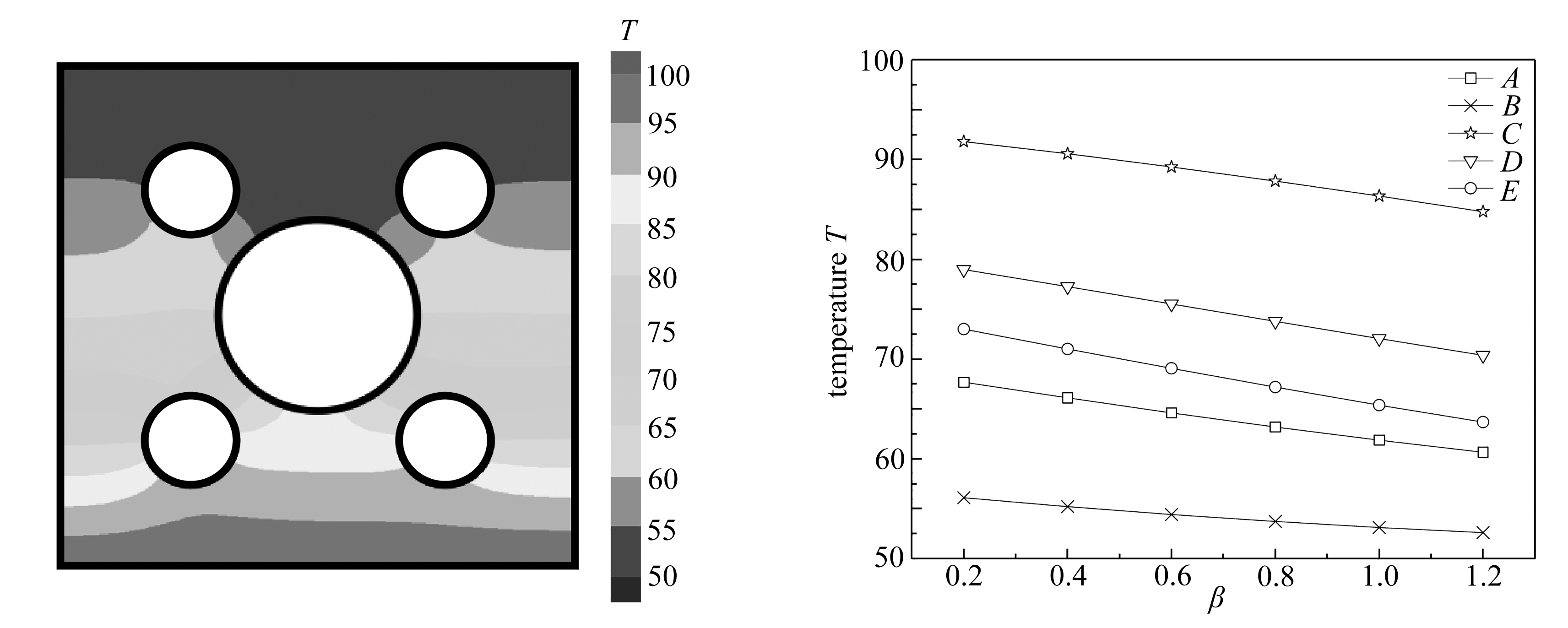Fig. 13 The temperature distribution for β=1 Fig. 14 Temperatures of measuring points at different graded parameters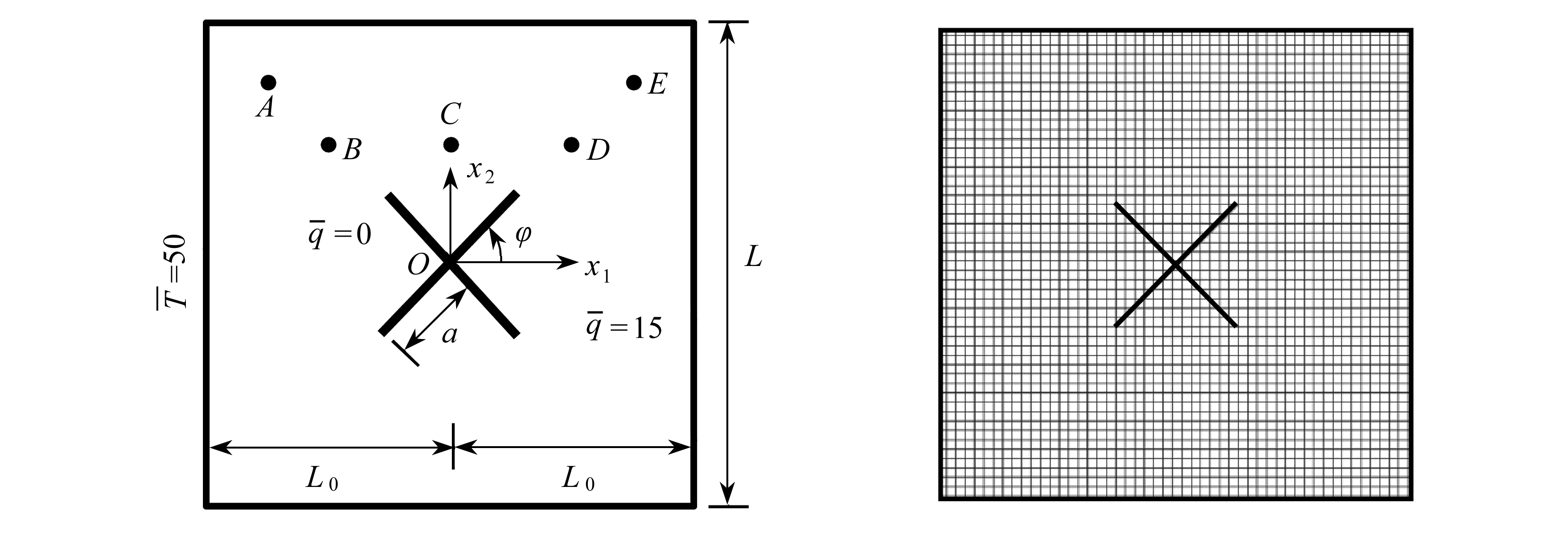Fig. 15 The physical model Fig. 16 The discretized domain for a=0.4 and φ=45°

NMM分析时， 取h=0.04首先研究裂纹长度对温度的影响φ=45°, a=0.2,0.3,0.4,0.5,0.6图16为a=0.4时的离散域，共包含2 784个物理分片和2 628个单元图17给出了相应温度场的分布情况，从图中可以明显地观察到温度场在穿越各裂纹面时的跳跃，这便直观展现了NMM可自然表征场函数在跨越裂纹时不连续的优点图18给出了五个测点：A(-0.75, 0.75)，B(-0.5, 0.5)，C(0.0, 0.5)，D(0.5, 0.5)和E(0.75, 0.75)的NMM模拟结果，容易看出各测点的温度均随裂纹长度的增加而增大，但点AB的温度变化不如其他点显著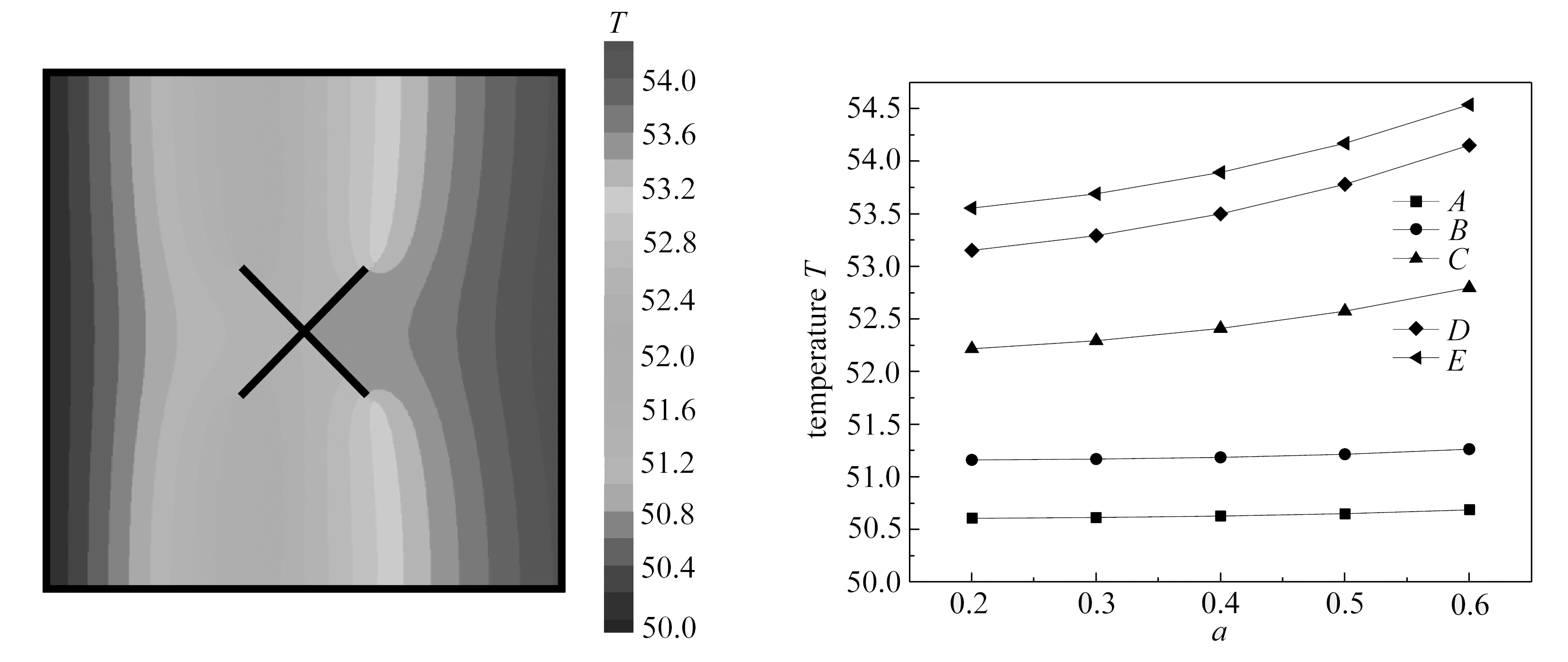Fig. 17 The temperature distribution for Fig. 18 Temperatures of measuring points a=0.4 and φ=45° at different crack lengths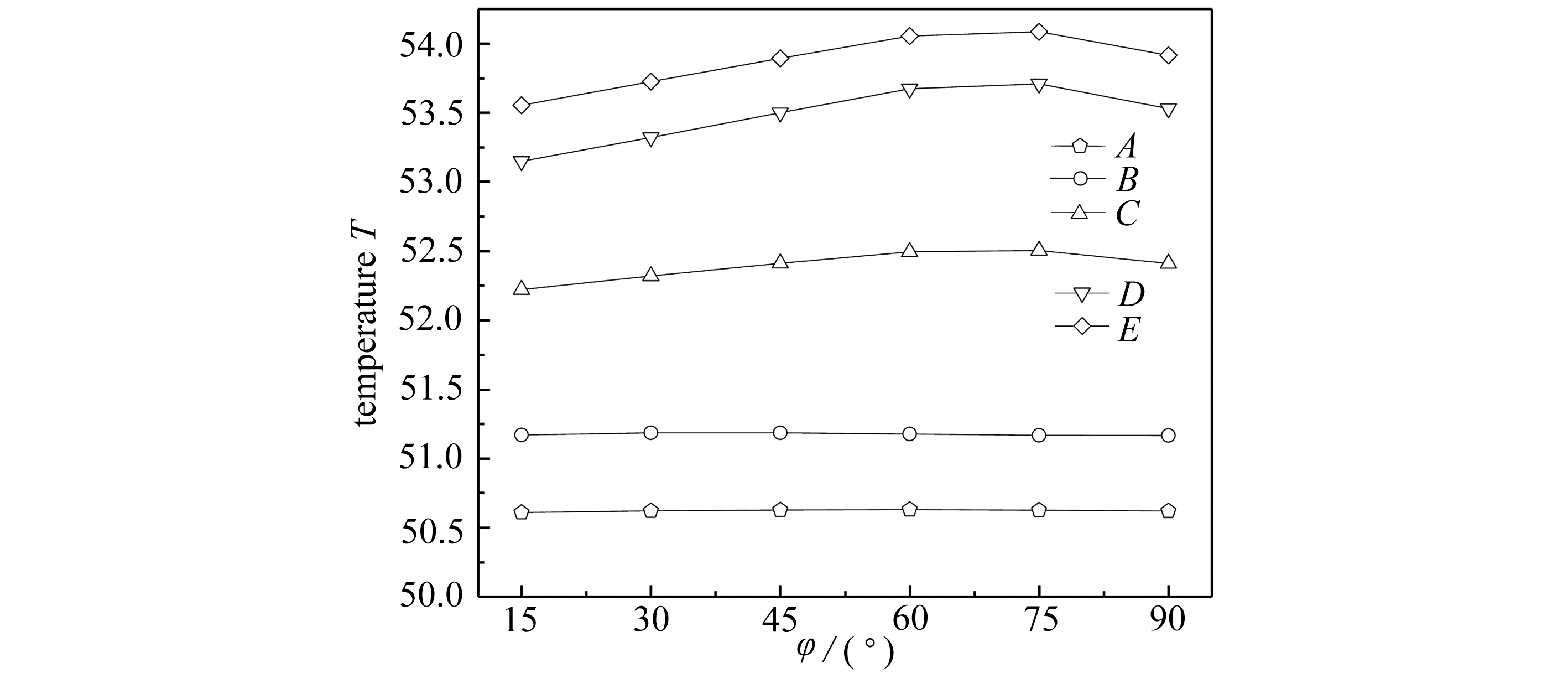Fig. 19 Temperatures of measuring points at different inclined angles

## 4 结 语

 PRESTINI D, FILIPPINI G, ZDANSKI P S B, et al. Fundamental approach to anisotropic heat conduction using the element-based finite volume method[J]. Numerical Heat Transfer, Part B: Fundamentals, 2017, 71(4): 327-345.

 闫相桥, 武海鹏. 正交各向异性材料三维热传导问题的有限元列式[J]. 哈尔滨工业大学学报, 2003, 35(4): 405-409. (YAN Xiangqiao, WU Haipeng. Finite element formulation of 3-D heat transfer in orthotropic materials[J]. Journal of Harbin Institute of Technology, 2003, 35(4): 405-409.(in Chinese))

 MERA N S, ELLIOTT L, INGHAM D B, et al. A comparison of boundary element method formulations for steady state anisotropic heat conduction problems[J]. Engineering Analysis With Boundary Elements, 2001, 25(2): 115-128.

 刘俊俏, 苗福生, 李星. 二维各向异性功能梯度材料热传导的边界元分析[J]. 西安交通大学学报, 2013, 47(5): 77-81.(LIU Junqiao, MIAO Fusheng, LI Xing. Boundary element analysis of the two-dimensional heat conduction equation for anisotropic fuctionally graded materials[J]. Journal of Xian Jiaotong University, 2013, 47(5): 77-81.(in Chinese))

 陈闽慷, 杜涛, 苏雪, 等. 二维非线性正交各向异性材料的瞬态热传导反问题数值方法[J]. 国防科技大学学报, 2017, 39(1): 194-198.(CHEN Minkang, DU Tao, SU Xue, et al. A numerical method for two-dimensional nonlinear transient inverse heat conduction problems for orthotropic material[J]. Journal of National University of Defense Technology, 2017, 39(1): 194-198.(in Chinese))

 谢佳萱, 李冬明, 聂峰华, 等. 正交各向异性稳态热传导问题的ICVEFG方法[J]. 固体力学学报, 2019, 40(1): 74-81.(XIE Jiaxuan, LI Dongming, NIE Fenghua, et al. The ICVEFG method for steady-state heat conduction problems in orthotropic media[J]. Chinese Journal of Solid Mechanics, 2019, 40(1): 74-81.(in Chinese))

 SHI G H. Manifold method of material analysis[C]//Proceedings of the 9th Army Conference on Applied Mathematics and Computing. Minneapolis, Minnesota, 1991.

 MA G W, AN X M, ZHANG H H, et al. Modeling complex crack problems using the numerical manifold method[J]. International Journal of Fracture, 2009, 156(1): 21-35.

 KOUREPINIS D, PEARCE C, BICANIC N. Higher-order discontinuous modeling of fracturing in concrete using the numerical manifold method[J]. International Journal of Computational Methods, 2010, 7(1): 83-106.

 YANG Y T, ZHENG H. A three-node triangular element fitted to numerical manifold method with continuous nodal stress for crack analysis[J]. Engineering Fracture Mechanics, 2016, 162: 51-75.

 WU Z J, JIANG Y L, LIU Q S, et al. Investigation of the excavation damaged zone around deep TBM tunnel using a Voronoi-element based explicit numerical manifold method[J]. International Journal of Rock Mechanics and Mining Sciences, 2018, 112: 158-170.

 WU Z J, XU X Y, LIU Q S, et al. A zero-thickness cohesive element-based numerical manifold method for rock mechanical behavior with micro-Voronoi grains[J]. Engineering Analysis With Boundary Elements, 2018, 96: 94-108.

 YANG S K, CAO M S, REN X H, et al. 3D crack propagation by the numerical manifold method[J]. Computers & Structures, 2018, 194: 116-129.

 YANG Y T, TANG X H, ZHENG H, et al. Hydraulic fracturing modeling using the enriched numerical manifold method[J]. Applied Mathematical Modelling, 2018, 53: 462-486.

 GUO H W, ZHENG H, ZHUANG X Y. Numerical manifold method for vibration analysis of Kirchhoff’s plates of arbitrary geometry[J]. Applied Mathematical Modelling, 2019, 66: 695-727.

 ZHENG H, YANG Y T, SHI G H. Reformulation of dynamic crack propagation using the numerical manifold method[J]. Engineering Analysis With Boundary Elements, 2019, 105: 279-295.

 林绍忠, 明峥嵘, 祁勇峰. 用数值流形法分析温度场及温度应力[J]. 长江科学院院报, 2007, 24(5): 72-75.(LIN Shaozhong, MING Zhengrong, QI Yongfeng. Thermal field and thermal stress analysis based on numerical manifold method[J]. Journal of Yangtze River Scientific Research Institute, 2007, 24(5): 72-75.(in Chinese))

 刘泉声, 刘学伟. 裂隙岩体温度场数值流形方法初步研究[J]. 岩土工程学报, 2013, 35(4): 635-642.(LIU Quansheng, LIU Xuewei. Preliminary research on numerical manifold method for temperature field of fractured rock mass[J]. Chinese Journal of Geotechnical Engineering, 2013, 35(4): 635-642.(in Chinese))

 谭育新, 张慧华, 胡国栋. 二维稳态热传导问题的正六边形流形元研究[J]. 应用数学和力学, 2017, 38(5): 594-604.(TAN Yuxin, ZHANG Huihua, HU Guodong. 2D steady heat conduction analysis with the regular hexagon numerical manifold method[J]. Applied Mathematics and Mechanics, 2017, 38(5): 594-604.(in Chinese))

 ZHANG H H, LIU S M, HAN S Y, et al. Modeling of 2D cracked FGMs under thermo-mechanical loadings with the numerical manifold method[J]. International Journal of Mechanical Sciences, 2018, 148: 103-117.

 ZHANG H H, LIU S M, HAN S Y, et al. The numerical manifold method for crack modeling of two-dimensional functionally graded materials under thermal shocks[J]. Engineering Fracture Mechanics, 2019, 208: 90-106.

 BABUSKA I, MELENK J M. The partition of unity method[J]. International Journal for Numerical Methods in Engineering, 1997, 40(4): 727-758.

 BAYESTEH H, AFSHAR A, MOHAMMDI S. Thermo-mechanical fracture study of inhomogeneous cracked solids by the extended isogeometric analysis method[J]. European Journal of Mechanics A: Solids, 2015, 51: 123-139.

 ZHANG H H, MA G W. Fracture modeling of isotropic functionally graded materials by the numerical manifold method[J]. Engineering Analysis With Boundary Elements, 2014, 38: 61-71.

 ZHANG H H, HAN S Y, FAN L F. Modeling 2D transient heat conduction problems by the numerical manifold method on Wachspress polygonal elements[J]. Applied Mathematical Modelling, 2017, 48: 607-620.

# Solutions of Continuous and Discontinuous Anisotropic Heat Conduction Problems With the Numerical Manifold Method

LIU Simin, ZHANG Huihua, HAN Shangyu, LIU Qiang

(School of Civil Engineering and Architecture, Nanchang Hangkong University, Nanchang 330063, P.R.China)

Abstract: The heat conduction is a common problem in engineering practice. Compared with those of isotropic materials, the heat conduction problem of anisotropic materials is more complicated, so it is of great significance to accurately predict the internal temperature distribution. The numerical manifold method (NMM) was developed to solve typical continuous and discontinuous heat conduction problems in anisotropic materials. According to the governing differential equation, boundary conditions and variational principles, the NMM discrete equations for such problems were derived. Several representative examples involving continuous and discontinuous situations were analyzed with the uniform mathematical cover independent of all physical boundaries. The results prove the feasibility and accuracy of the method and indicate that the NMM can simulate the heat conduction problem of anisotropic materials well. Besides, the influences of the material properties and crack configurations on the temperatures were also investigated.

Key words: anisotropic; steady heat conduction; numerical manifold method; temperature; continuous and discontinuous problem

*收稿日期： 2019-09-25； 修订日期：2019-10-25

DOI: 10.21656/1000-0887.400289

Foundation item: The National Natural Science Foundation of China(11462014)